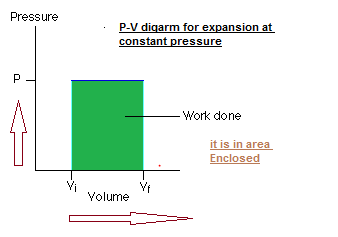Home » Physics » Thermodynamics Problem solving tips

# Thermodynamics Problem solving tips

[latexpage]

## Thermodynamics tips and tricks

Thermodynamics is very important topic that you want to learn completely whether you are appearing for your school exams or for competitive exams like IITJEE or PMT. Thermodynamics is considered fairly easy but this does not mean you can take it lightly. Take your time in mastering concepts of thermodynamics so you can solve thermodynamics problems easily

1. Net Heat flow at a junction is Zero
2. Internal Energy does not depend on the path
3. Internal Energy in a cyclic Process is zero

4. Work done is given by the area enclosed by the process on the PV diagram5. Always make sure you Heat(Q) ,Internal Energy(U) and Work(W) with correct sign in equation $U=Q-W$

W is positive when work done by the system(Increase in Volume)

W is negative when work done on the system(Decrease in Volume)

Q is positive when heat is given to the system

Q is negative when heat is taken from the system
6. Change in Internal energy is zero for a cycle , So Net Heat=Net Work done
7. All Bodies emit radiation at all temperature.Radiation emitted are of different frequencies.Frequency at which most radiation are emitted are directly proportional to Temperature of the body.

Isothermal Process

In isothermal process temperature of the system remains constant throughout the process.

For an iso-thermal process equation connecting P, V and T gives.

PV = constant

$VdP+PdV=0$ , $– \frac{VdP}{dV} =P$

Now Bulk Modulus$= -\frac{VdP}{dV}$

Work-Done: W=nRT ln(V2/V1)

Process in which no heat enters or leaves a system is called an adiabatic process

$PV^{y}=constant$

$V^{y}dP+P^{y}V^{y-1}dV=0$

Workdone: (P1V1-P2V2)/(?-1)

if A sold sphere and a hollow sphere having same material and size are heated to same temperature and allowed to cool in the same surrounding ,it has been found that hollow sphere cools faster than solid sphere

Isochoric process:
• In an isochoric process volume of the system remain uncharged throughout i.e. ?V=0.
• When volume does not change, no work is done; ?W = 0 and therefore from first law
U2 – U1 = ?U =?Q
• All the heat given to the system has been used to increase the internal energy of the system.

Isobaric Process:
• A process taking place at constant pressure is called isobaric process.
• From equation (3) we see that work done in isobaric process is
W=P(V2-V1)=nR(T2-T1)
where pressure is kept constant.
• Here in this process the amount of heat given to the system is partly used in increasing temperature and partly used in doing work

### Thermodynamics book for class 11 and competitive exams

[/iframe]

Subscribe
Notify of

This site uses Akismet to reduce spam. Learn how your comment data is processed.